## Towards the implementation of a vector data type in GIS

In contrast to scalars, which only have magnitude, vectors are geometrical objects defined by a magnitude and a direction. They play an important role in physics, since they can represent the forces acting on a body, velocities and accelerations, etc.

In Geographic Information Science (GIS) analysis we are used to work with scalar fields, h(x,y), which are continuous differentiable functions which associate a scalar to every point in space, normally using a fixed spatial structure called a grid. Similarly, a vector field f(x,y) is defined as a continuous differentiable function which associates a vector to every point in space. The temperature of the air over a region is an example of a scalar field, whereas the direction and magnitude of the wind is a vector field. The values of a scalar field h(x,y) are usually represented by a continuous grey or color scale, or alternatively by contour lines. A vector field f(x,y) can be represented by arrows with origin in the center of the grid cells, and longitude proportional to the vector modulus. Most GIS packages provide complete toolsets for representing and operating scalar fields, but lack a specific data type and the associated tools for representing and operating vector fields (Figure 1).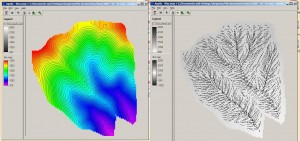Figure 1. Graphical representation of a scalar field (left, elevation above sea level) and a vector field (right, slope gradient).

Although all GIS allow correct representation of scalar fields, as far as I know no one provides a convenient representation for vector fields, so the most intuitive representation of vectors, such as the one shown in Figure 1, is not possible. From an operative point of view, vectors in a 2‑dimensional Euclidean space can be represented in a Cartesian system by the coordinates of the origin and the end point. In a grid GIS, in which the origin points are given by the spatial structure of the grid mesh, only the endpoints need to be recorded. These can be done by a pair of real numbers, sometimes called a row vector or column vector (Figure 2).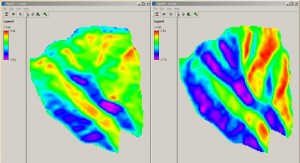Figure 2. Representation of the vector field in Figure 1 by decomposing it into its x‑wise (left) and y‑wise (right) components.

Each vector in Figure 1 is decomposed into its x- and y‑wise components, i.e. two scalars, which need to be represented separatedly. The sign of the components indicates the direction, e.g. to the right or left in the case of the x‑wise component and up or down in the case of the y‑wise component. The meaning of the positive and negative values is conventional and must be defined in advance.

Operations on vector data

Algebraic operations such as addition, subtraction and multiplication (dot product and cross product) can be performed on vectors. Addition of vectors, for example, is common when combining forces from different sources acting on a mass. Vector operations affect both the direction and magnitude of the vectors.

Scalar multiplication is another useful vector operation, in which the modulus of the vector is multiplied by a scalar value. For example, the force acting on a body is obtained by the scalar multiplication of a mass (a scalar) and an acceleration (a vector).

Some operators from vector calculus can also be very useful for GIS vector analysis. These are the gradient, curl, divergence and laplacian operators.

The gradient operator measures the rate and direction of change in a scalar field. It maps a scalar field to a vector field, and is defined as: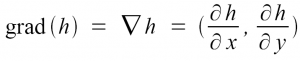The symbol (nabla) is also called the gradient or del operator. The map on the right hand side of Figure 1 represents the gradient of the elevation map shown on the left.

The curl operator measures the tendency to rotate about a point in a vector field. It maps a vector field to another vector field, and is defined as: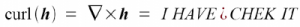The symbol (nabla cross) is also called the rotor or rot operator.

The divergence operator measures the magnitude of a source or sink in a vector field. It maps a vector field to a scalar field, and is defined as: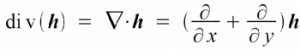The result of the divergence operator is a signed scalar field representing the change in the vector field at any given point (Figure 3). The divergence operator is used for example in mass and momentum conservative models.

The laplacian operator measures the divergence of the gradient of a scalar field (what is commonly known as the curvature in GIS analysis; Figure 4). It maps a scalar field to a scalar field, and is a composition of the divergence and the gradient operators: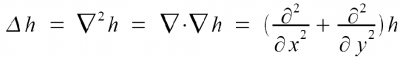Examples of vector operations

An example of a model that can be expressed in terms of the previous vector operators is the advection equation, which describes the transport of a preperty represented by a scalar d in a velocity field u: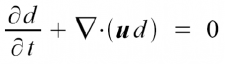The previous equation describes transport in a fluid, for example the transport of pollutants downstream a river. The fluid is conveniently described as a vector field, and the concentration of the material being transported is represented by a scalar. In a solenoidal vector field (that is, nabla cdot {bold u} = 0), the previous equations is simplified as: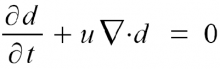Another example is the diffusion equation, which describes the dispersion in a scalar field as rising from Brownian motion (random walk) of particles which at the macroscopic scale results in motion from high to low concentration areas. The diffusion equation is defined as: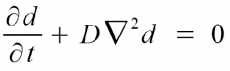where D is a diffusion coefficient. The above equation can describe the spreading of smoke in the air, or the dispersion of a contaminant from a point source in a lake.